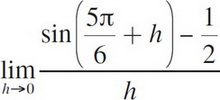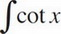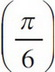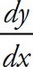# AP Calculus AB Practice Test 15

### Test Information7 questions21 minutes

Calculator Allowed

1.=

2. The base of a solid S is the region enclosed by the graph of 4x + 5y = 20, the x-axis, and the y-axis. If the cross-sections of S perpendicular to the x-axis are semicircles, then the volume of S is

3. Which of the following is an equation of the line tangent to the graph of y = x3 + x2 at y = 3 ?

4. If f′(x) = ln x - x + 2, at which of the following values of x does f have a relative minimum value?

5. Find the total area of the region between the curve y = cos x and the x-axis from x = 1 to x = 2 in radians.

6. Let f(x) =dx; 0 < x < π. If f= 1, then f(1) =

7. A radioactive isotope, y, decays according to the equation= ky, where k is a constant and t is measured in seconds. If the half-life of y is 1 minute, then the value of k is